## Flipflop stationary state

exa11
Variables and parameters:

y1,y2: Voltages in inverter 1 (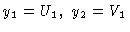)

y3,y4: Voltages in inverter 2 ()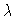: voltage supply

System of equations: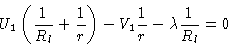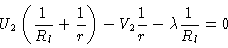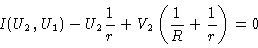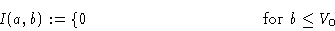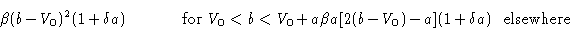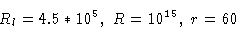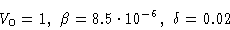Figure 1
Two identical inverters coupled to a flipflop

Figure 2
Bifurcation diagram y1 versus.

Figure 3
(y1,y3) phase diagram for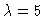.

Figure 4
(y1,y3) phase diagram for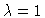.

This document
Figure 1
Figure 2
Figure 3
Figure 4